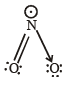Courses

# Test: 35 Year JEE Previous Year Questions: Chemical Bonding & Molecular Structure

## 34 Questions MCQ Test Class 11 Chemistry 35 Years JEE Mains &Advance Past yr Paper | Test: 35 Year JEE Previous Year Questions: Chemical Bonding & Molecular Structure

Description
This mock test of Test: 35 Year JEE Previous Year Questions: Chemical Bonding & Molecular Structure for JEE helps you for every JEE entrance exam. This contains 34 Multiple Choice Questions for JEE Test: 35 Year JEE Previous Year Questions: Chemical Bonding & Molecular Structure (mcq) to study with solutions a complete question bank. The solved questions answers in this Test: 35 Year JEE Previous Year Questions: Chemical Bonding & Molecular Structure quiz give you a good mix of easy questions and tough questions. JEE students definitely take this Test: 35 Year JEE Previous Year Questions: Chemical Bonding & Molecular Structure exercise for a better result in the exam. You can find other Test: 35 Year JEE Previous Year Questions: Chemical Bonding & Molecular Structure extra questions, long questions & short questions for JEE on EduRev as well by searching above.
QUESTION: 1

### In which of the following species the interatomic bond angle is 109° 28’? 

Solution:

Both NH3​ and (BF4​)-1 have same hybridisation, sp3, we know in NH3​, there is a lone pair, which makes the bond angle 109°28′.

(BF4​)-1 and NH3​ has pyramidal structure. So they have same bond angle.

QUESTION: 2

### Which of the following are arranged in an increasing order of their bond strengths? 

Solution: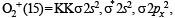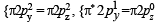Bond order =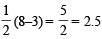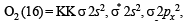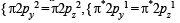Bond order =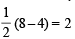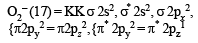Bond order =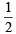(8 – 5) =  1.5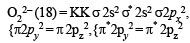Bond order =(8 –6 ) =  1

NOTE : As we know that as the bond order decreases, stability also decreases and hence the bond strength also decreases. Hence the correct order of their increasing bond strength is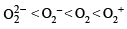QUESTION: 3

### Hybridisation of the underline atom changes in: 

Solution: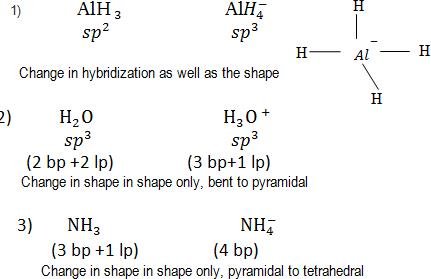QUESTION: 4

An ether is more volatile than an alcohol having the same molecular formula. This is due to 

Solution:

There is inter-molecular H binding in alcohols, thus giving them higher boiling point and lower volatility.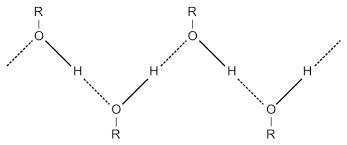Hence C is correct.

QUESTION: 5

Which one of the following pairs of molecules will have permanent dipole moments for both members ? 

Solution:

Both NO2 and O3 have angular shape and hence will have net dipole moment.

QUESTION: 6

Which one of the following compounds has the smallest bond angle in its molecule ? 

Solution:

In H2S, due to low electronegativity of sulphur the L.P. - L. P. repulsion is more than B. P. - B. P. repulsion and hence the bond angle is minimum.

SO2    H2O   H2S    NH3
Bond angle 119.5° 104.5° 92.5° 106.5°

QUESTION: 7

The pair of species having identical shapes for molecules of both species is 

Solution:

Both XeF2 and CO2 have a linear structure.
F — Xe — F O = C = O

QUESTION: 8

The correct order of bond angles (smallest first) in H2S, NH3, BF3 and SiH4 is 

Solution:

The order of bond angles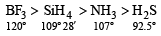QUESTION: 9

The bond order in NO is 2.5 while that in NO+ is 3. Which of the following statements is true for these two species ? 

Solution:

Now sin ce bond order of NO+ given (3) is higher than that of NO (2.5). Thus bond length of NO+ will be shorter.

QUESTION: 10

The states of hybridization of boron and oxygen atoms in boric acid (H3BO3) are respectively 

Solution: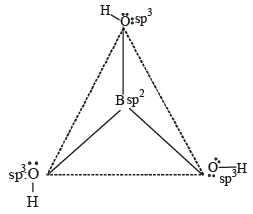QUESTION: 11

Which one of the following has the regular tetr ahedral structure ? 

(Atomic nos. : B = 5, S = 16, Ni =28, Xe = 54)

Solution:

XeF4 (sp3d2 square planar),
[ Ni(CN)4 ]2- (dsp2 square planar ),
BF4- (sp3 tetrahedral), SF4 (sp3d see saw shaped)

QUESTION: 12

The maximum number of 90º angles between bond pair-bond pair of electrons is observed in 

Solution: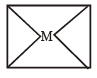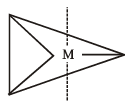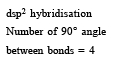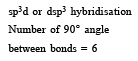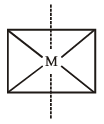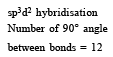QUESTION: 13

Lattice energy of an ionic compound depends upon

Solution:

The value of lattice energy depends on the charges present on the two ions and the distance between them.

QUESTION: 14

Which of the following molecules/ions does not contain unpaired electrons? 

Solution:

The distribution of electrons in MOs is as follows :

N2+(electrons 13)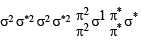O2 (electrons 16)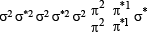O22– (electrons 18)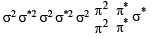B2 (electrons 10)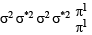Only O22– does not contain any unpaired electron.

QUESTION: 15

In which of the following molecules/ions are all the bonds not equal? 

Solution:

In SF4 the hybr idisation is sp3d and the shape of molecule is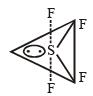QUESTION: 16

The decreasing values of bond angles from NH3 (106º) to SbH3 (101º) down group-15 of the periodic table is due to 

Solution:

The bond angle decreases on moving down the group due to decrease in bond pair-bond pair repulsion.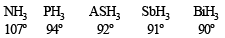NOTE : This can also be explained by the fact that as the size of central atom increases sp3 hybrid orbital becomes more distinct with increasing size of central atom i.e. pure p- orbitals are utilized in M–H bonding

QUESTION: 17

Which of the following species exhibits the diamagnetic behaviour ? 

Solution:

Diamagnetic species have no unpaired electrons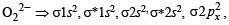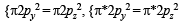Whereas paramagnetic species has one or more unpaired electrons as in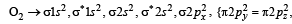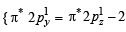unpaired electrons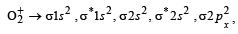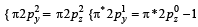unpaired electron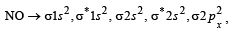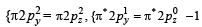unpaired electron

QUESTION: 18

The charge/size ratio of a cation determines its polarizing power. Which one of the following sequences represents the increasing order of the polarizing power of the cationic species, K+, Ca2+, Mg2+, Be2+? 

Solution:

Smaller the size and higher the charge more will be polarising power of cation. Since the order of the size of cati on is K+ > Ca++ > Mg ++> Be++ . So the correct order of polarising power is K+ < Ca2+ < Mg2+ < Be2+

QUESTION: 19

In which of the following ionization processes, the bond order has increased and the magnetic behaviour has changed? 

Solution:

(a)N2 : bond order 3, paramagnetic
N2 : bond order, 2.5, paramagnetic
(b) C2 : bond order 2, diamagnetic
C2+ : bond order 1.5, paramagnetic
(c) NO : bond order 2.5, paramagnetic
NO+ : bond order 3, diamagnetic
(d) O2 : bond order 2, paramagnetic
O2+ : bond order 2.5, paramagnetic

QUESTION: 20

Which of the following hydrogen bonds is the strongest? 

Solution:

NOTE : Greater the difference between electronegativity of bonded atoms, stronger will be bond. Since F is most electronegative hence F – H ...... F is the strongest bond.

QUESTION: 21

Which one of the following pairs of species have the same bond order? 

Solution:

For any species to have same bond order we can expect them to have same number of electrons. Calculating the number of electrons in various species.
O2 (8 + 8 +1= 17) ; CN- (6 + 7 +1= 14)
NO+(7 + 8 – 1= 14); CN+ (6 + 7 –1 = 12)
We find CN and NO+ both have 14 electrons so they have same bond order. Correct answer is (a).

QUESTION: 22

The bond dissociation energy of B – F in BF3 is 646 kJ mol–1 whereas that of  C – F in CF4 is 515 kJ mol–1. The correct reason  for higher B – F bond dissociation energy as compared to that of C – F is 

Solution:

NOTE : The delocalised pπ -pπ bonding between filled p-orbital of F and vacant p-orbital of B leads to shortening of B–F bond length which results in higher bond dissociation energy of the B–F bond.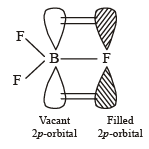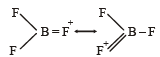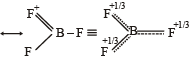QUESTION: 23

Using MO theory, predict which of the following species has the shortest bond length? 

Solution:

Bond orderBond order in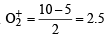Bond order in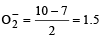Bond order in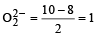Bond order in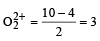Since Bond order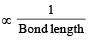∴  Bond length is shortest in O22+ .

QUESTION: 24

Among the following the maximum covalent character is shown by the compound 

Solution:

The proportion of covalent character in an ionic bond is decided by polarisability of the metal cation as well as the electrongativity of both elements involved in bonding. Polarisability is further decided by the density of positive charge on the metal cation. AlCl3 is considered to show maximum covalent character among the given compounds. This is because Al3+ bears 3 unit of positive charge and shows strong tendency to distort the electron cloud, thus the covalent character in Al-Cl bond dramatically increases.

QUESTION: 25

The hybridization of orbitals of N atom in NO3 , NO2+ and NH4+ are respectively : 

Solution:

The formula to find the hybridisation of central atom is
Z =[Number of valence electrons on central atom +No. of monovalent  atom altached to it + negative charge if any – positive charge if any]
For NO– Z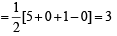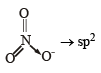For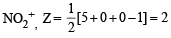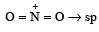For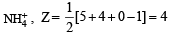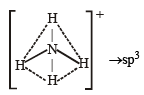QUESTION: 26

The structure of IF7 is 

Solution:

Pentagonal bipyramidal shape.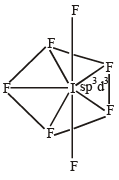QUESTION: 27

Ortho-Nitrophenol is less soluble in water than p- and mNitrophenols because : 

Solution:

Compounds involved in chelation become non-polar.
Consequently such compounds are soluble in nonpolar solvents like ether, benzene etc. and are only sparingly soluble in water whereas meta and para isomers are more soluble in water & less soluble in non-polar solvents.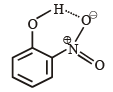intra-molecular H-bonding

QUESTION: 28

In which of the following pairs the two species are not isostructural ? 

Solution:

PF5 trigonal bipyramidal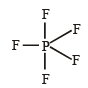BrF5 square pyramidal (distorted)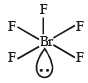*Multiple options can be correct
QUESTION: 29

Which one of the following molecules is expected to exhibit diamagnetic behaviour ? [JEE M 2013]

Solution:

The molecular orbital structures of C2 and N2 are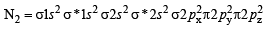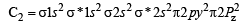both N2 and C2 have paired electrons in its molecular orbital hence are diamagnetic.

QUESTION: 30

Which of the following is the wrong statement ? [JEE M 2013]

Solution:

(a)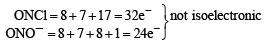(b)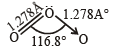The central atom is sp2 hybridized with one lone pair.

(c) It is a pale blue gase. At – 249.7°, it forms violet black crystals.

(d) It is diamagnic in nature due to presence of paired electrons.

QUESTION: 31

In which of the following pairs of molecules/ions, both the species are not likely to exist ? [JEE M 2013]

Solution: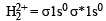bond order for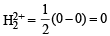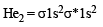bond order for He2 =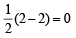so both H22+ and He2 does not exist

QUESTION: 32

Stability of the species Li2, Li2- and Li2+ increases in the order of : [JEE M 2013]

Solution: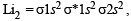∴ Bond order =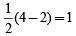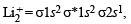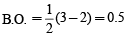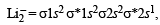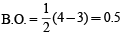The bond order of Li2+ and Li2 is same but Li2+ is more stable than Li2 because Li2+ is smaller in size and has 2 electrons in Anti bonding orbital whereas Li2 has 3 electrons in Anti bonding orbital. hence Li2+ is more stable than Li2

QUESTION: 33

For which of the following molecule significant μ ≠ 0? [JEE M 2014]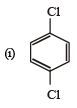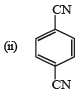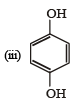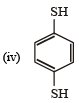Solution: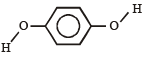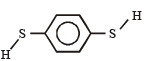In both the molecules the bond moments are not canceling with each other and hence the molecules has a resultant dipole and hence the molecule is polar.

QUESTION: 34

The species in which the N atom is in a state of sp hybridization is : [JEE M 2016]

Solution:

Hybridization (H) =[no. of valence electrons of central atom + no. of Monovalent atoms attached to it + (–ve charge if any) – (+ve charge if any)]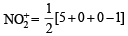= i.e. sp hybridisation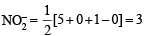i.e. sp2 hybridisation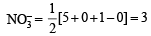i.e. sp2 hybridisation

The lewis structure of NO2 shows a bent molecular geometry with trigonal planar electron pair geometry hence the hybridization will be sp2